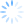Distance calculations are put in use when estimating freight costs, identifying closest customers and for many other business purposes. One popular application is a radius analysis, using distance to determine the nearest customers in a list to a central location. For convenience, most calculations use distance between zip codes.

Calculation of the distance between zip codes is based upon latitude and longitude information for each zip code. This geocoding data is determined by calculating the centroid of boundaries of each zip code. You can think of a centroid as a balance point, a location near the center of the areas of a polygon that make up the zip code.

CDXZipStream and other software used for distance and radius analysis use this geocoding information to calculate a straight line distance to a chosen zip code. All possible combinations of zip codes are reviewed and then sorted in ascending distance to show the closest ones. This type of analysis is fine when trying to point a customer to your nearest location, and every location is in a separate zip code.

But you should be aware of the possible inaccuracies. The land area in many zip codes can be hundreds of square miles. In fact the largest one in Tonopah, Nevada is 5,496 square miles. So if a site is on the periphery of a zip code distance calculations can be many miles in error. If you would like to know the land area associated with your zip code you can find this in our demographic database, along with water area and hundred of other items.

So what do you do if you need a more precise analysis? The solution is to determine exact locations by address, city and state for all locations. With this latitude and longitude information you can then determine the true, not approximated distance. CDXZipStream has a straight line calculation, CDXDistance2WP, to calculation distance between locations. CDXRouteMP can be used to calculate driving distance between addresses. So even evaluating multiple sites in the same zip code is no longer a problem. We’ll go into more detail on doing this in a future article.